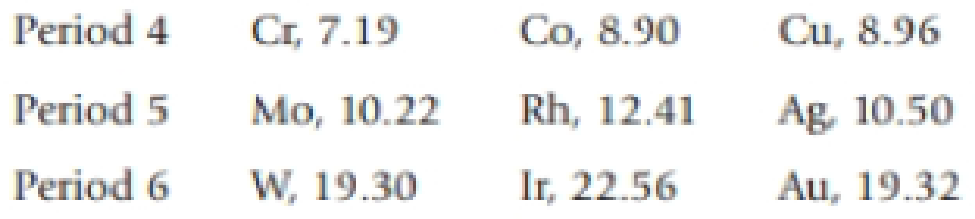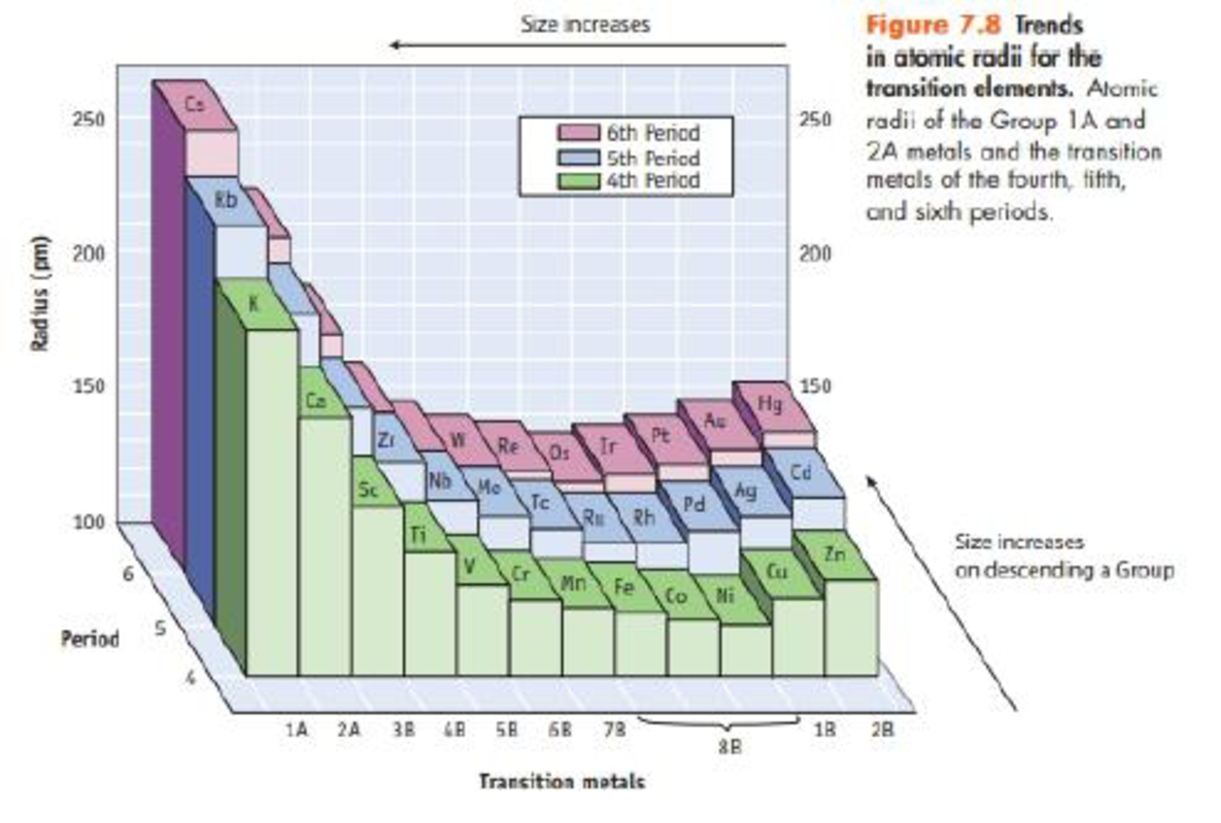Chapter 7, Problem 82SCQ

Chapter
Section
Textbook Problem

The densities (in g/cm3) of elements in Croups 6B, 8B, and 1B are given in the table below.Transition metals in the sixth period all have much greater densities than the elements in the same groups in the fourth and fifth periods. Refer to Figure 7.8, and explain this observation.Interpretation Introduction

Interpretation:

The sixth period transition metals has greater density than other groups, the reason has to be explained.

Concept Introduction:

Atomic radius: The concept explain chemical element is a measured of the size of its atoms, in other words to measure the distance from the center of the nucleus to the boundary of the surrounding cloud of number of electrons.

Density properties of transition metals: Generally the transition metals possess a high density, high melting points and high boiling points. These properties are due to metallic bonding by delocalized (d-electrons) and leading to cohesion which increases with the number of shared electrons.

Explanation

As we know the general formula of density,

Density=MassVolume

From the figure, the nuclear volume of the transition elements is much lower than those s- and p-block elements; is due to the filling of

Still sussing out bartleby?

Check out a sample textbook solution.

See a sample solution

The Solution to Your Study Problems

Bartleby provides explanations to thousands of textbook problems written by our experts, many with advanced degrees!

Get Started

What is the definition of science?

An Introduction to Physical Science

Distinguish between acids and bases. What are their properties?

Biology: The Dynamic Science (MindTap Course List)

Compare the shapes of unhybridized p and hybridized sp3 orbitals.

Chemistry for Today: General, Organic, and Biochemistry